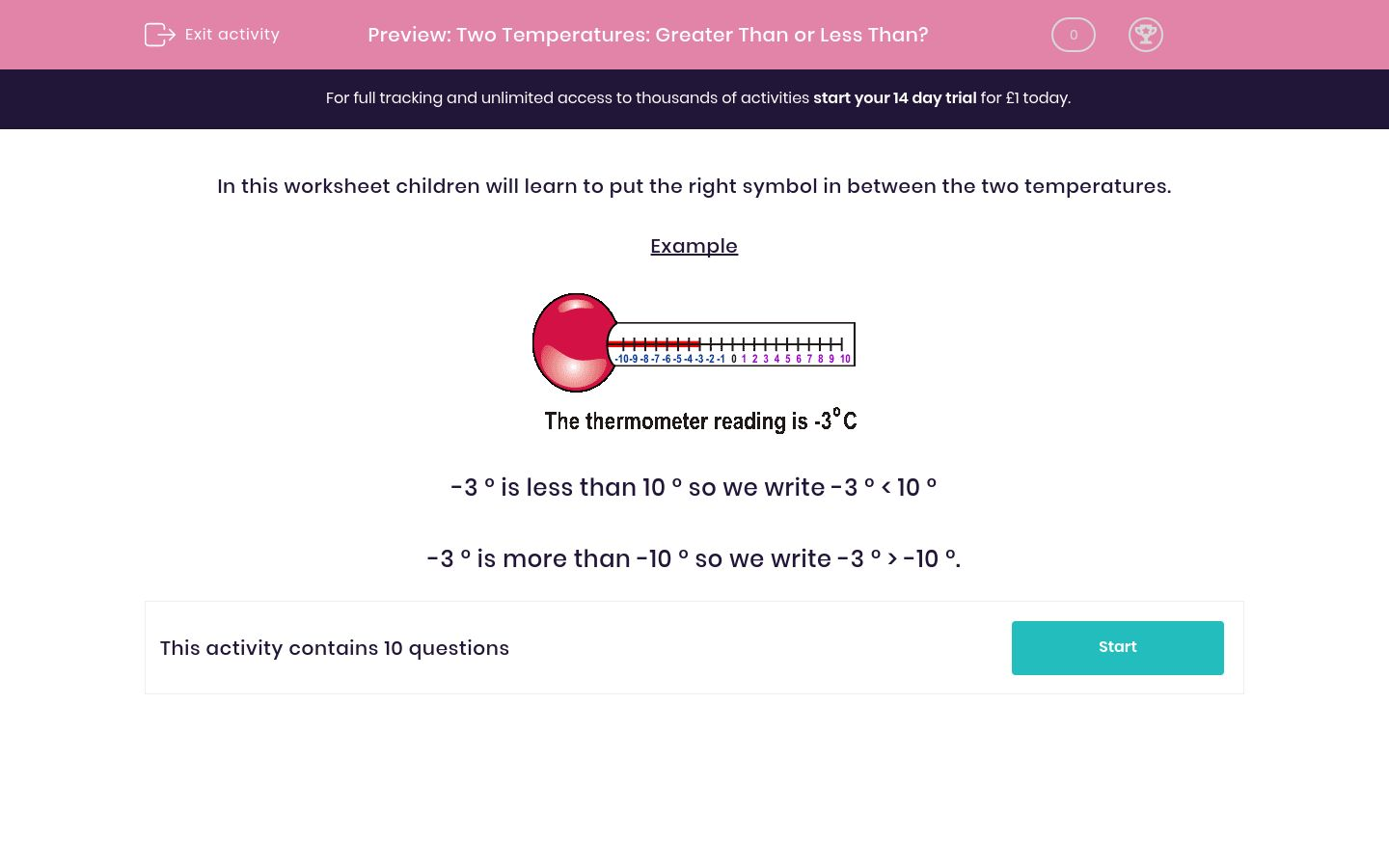# Two Temperatures: Greater Than or Less Than?

In this worksheet, students look at a number (which may be negative) and decide whether the number that follows it is greater than (>) or less than (<) the first number.Key stage:  KS 2

Curriculum topic:   Maths and Numerical Reasoning

Curriculum subtopic:   Place Value

Difficulty level:### QUESTION 1 of 10

In this worksheet children will learn to put the right symbol in between the two temperatures.

Example-3 ° is less than 10 ° so we write -3 ° < 10 °

-3 ° is more than -10 ° so we write -3 ° > -10 °.

Put the right symbol > or < between the two numbers.

0 ° -9 °
<

>

Put the right symbol > or < between the two numbers.

-5° 9 °
<

>

Put the right symbol > or < between the two numbers.

-3° -7°
<

>

Put the right symbol > or < between the two numbers.

-3° -10°
<

>

Put the right symbol > or < between the two numbers.

-9° -5°
<

>

Put the right symbol > or < between the two numbers.

<

>

Put the right symbol > or < between the two numbers.

-10°

<

>

Put the right symbol > or < between the two numbers.

-9°
<

>

Put the right symbol > or < between the two numbers.

-7°
<

>

Put the right symbol > or < between the two numbers.

-8°  6°
<

>

• Question 1

Put the right symbol > or < between the two numbers.

0 ° -9 °
>
• Question 2

Put the right symbol > or < between the two numbers.

-5° 9 °
<
• Question 3

Put the right symbol > or < between the two numbers.

-3° -7°
>
• Question 4

Put the right symbol > or < between the two numbers.

-3° -10°
>
• Question 5

Put the right symbol > or < between the two numbers.

-9° -5°
<
• Question 6

Put the right symbol > or < between the two numbers.

>
• Question 7

Put the right symbol > or < between the two numbers.

-10°

<
• Question 8

Put the right symbol > or < between the two numbers.

-9°
<
• Question 9

Put the right symbol > or < between the two numbers.

-7°
>
• Question 10

Put the right symbol > or < between the two numbers.

-8°  6°
<
---- OR ----

Sign up for a £1 trial so you can track and measure your child's progress on this activity.

### What is EdPlace?

We're your National Curriculum aligned online education content provider helping each child succeed in English, maths and science from year 1 to GCSE. With an EdPlace account you’ll be able to track and measure progress, helping each child achieve their best. We build confidence and attainment by personalising each child’s learning at a level that suits them.

Get started